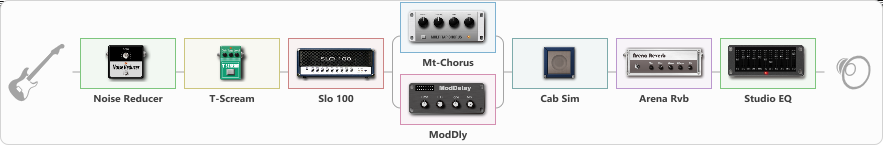# Maneskin

Discussion in 'ToneLib-GFX presets' started by truss van halen, Mar 10, 2021.

1. ### truss van halenWell-Known Member

Maneskin

Preset name: Maneskin

Effects chain:Effect: "Noise Reducer" (Dynamics / Filter), active - "yes"
{
"Sens" = 100
"Mode" = Hard
}

Effect: "T-Scream" (Overdrive / Distortion), active - "yes"
{
"Drive" = 0
"Tone" = 50
"Level" = 61
}

Effect: "Slo 100" (Amp simulators), active - "yes"
{
"Gain" = 24
"Bass" = 49
"Middle" = 63
"Treble" = 41
"Presence" = 50
"Master" = 69
"Level (dB)" = 0
}

Effect: "Splitter" (Dynamics / Filter)
{
"A-Bypass" = Off
"A-Pan" = 0
"A-Level" = 55
"B-Bypass" = Off
"B-Pan" = 0
"B-Level" = 55

'A' branch:
{

Effect: "Mt-Chorus" (Modulation / Sfx), active - "yes"
{
"Speed" = 3.1
"Depth" = 69
"Time" = 3.4
"Mix" = 61
}
}
'B' branch:
{

Effect: "ModDly" (Delay), active - "yes"
{
"Time" = 344
"Feedback" = 10
"Tone" = 70
"Speed" = 1.7
"Mix" = 50
}
}
}

Effect: "Cab Sim" (Cabinets), active - "yes"
{
"Model" = 4x12" Greenback
"Level (dB)" = 0
}

Effect: "Arena Rvb" (Reverberation), active - "yes"
{
"Time" = 3.0
"PreDelay" = 3
"LoDamp" = 18
"HiDamp" = 21
"Mix" = 43
}

Effect: "Studio EQ" (Dynamics / Filter), active - "yes"
{
"31 Hz" = 0
"62 Hz" = 0
"125 Hz" = 8
"250 Hz" = 0
"500 Hz" = 4
"1 kHz" = 0
"2 kHz" = 0
"4 kHz" = 6
"8 kHz" = 0
"16 kHz" = 0
"Level (dB)" = 0
}

Note: You will need to download and install the ToneLib-GFX software to use the preset.

File size:
866 bytes
Views:
2,530